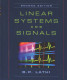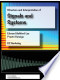### Signals and Systems

#### Course Description

In this course a computational view of signals and systems is implemented and students will acquire basic knowledge necessary in computer engineering, communication, control and electronics. Topics are as follows. Signals as functions. Discrete and continuous time signals. Elementary signal operations. Elementary signals. The four Fourier transforms: CTFS, CTFT, DTFS, DTFT. Fourier transform properties. Sampling and discrete-time signal processing. Discrete time Fourier transform. Systems as functions. Systems with the memory. Linear and time invariant (LTI) systems. Convolution sum and convolution integral. Response of linear time invariant systems. Transfer functions and frequency response. Laplace and z-transform in LTI system analysis. State-space model. Basic structures for the implementation of LTI systems.

#### General Competencies

Students will learn and understand basis of description and analysis of discrete and continuous-time signals and systems. They will also obtain practical skills in system analysis in MATLAB.

#### Learning Outcomes

1. classify signals;
2. explain and apply tests to unknown systems in order to classify the systems into known categories;
3. explain and compare the properties of the Fourier Transform, the Laplace Transform and the Z-Transform;
4. apply methods to find response of LTI time discrete and time continuous systems in time domain;
5. apply Laplace and z- transform to find response of LTI time discrete and time continuous systems;
6. analyze transfer functions and frequency responses and present the properties of the systems;
7. analyze MIMO systems described with state equations;
8. analyze and implement linear systems using block diagrams.

#### Forms of Teaching

Lectures

Course is divided in two cycles of lecturing. There are six weeks in the first cycle, and seven weeks lecturing in second cycle. Two weeks are reserved for midterm and final exam.

Exams

Midterm and final exam are organized as written exams.

Exercises

Optional recitation sessions are offered weekly with the duration of two hours.

Consultations

Lecturers in charge:

Lecturers:

• Doc. dr. sc. Marko Subašić, consultations immediately after lectures
• Dr. sc. Ana Sović, consultations immediately after lectures and Fridays in B4 at 12:00

Teaching assistants

Other Forms of Group and Self Study

Homework, available on Moodle.

Continuous Assessment Exam
Laboratory Exercises 0 % 6 % 0 % 0 %
Homeworks 0 % 10 % 0 % 0 %
Class participation 0 % 4 % 0 % 0 %
Mid Term Exam: Written 50 % 40 % 0 %
Final Exam: Written 50 % 40 %
Exam: Written 51 % 100 %

#### Week by Week Schedule

1. Signals as functions. Introduction, motivation, organization of the course. Introduction to signals and systems. Signals as functions. Classification of signals. Elementary signal operations. Signal energy and signal power.
2. Elementary signals: unit step, unit ramp, Kronecker delta function, Dirac delta function. Generalized derivative of signals with jump discontinuities. Continuous and discrete time sinusoid and complex exponential signal.
3. Fourier transforms: CTFS and CTFT. Generalized Fourier transform. Fourier transform of Dirac delta function.
4. Fourier transforms: DTFS and DTFT. CTFT and DTFT of periodic signals.
5. Fourier transform properties: linearity, symmetry, convolution, time and frequency shift, duality. Continuous time signal sampling. Sampling theorem. Aliasing. Reconstruction of a signal from its samples.
6. Continuous spectrum sampling. Continuous spectrum reconstruction from its samples. Discrete Fourier transform – DFT.
7. midterm
8. Systems as functions. Instantaneous and dynamic systems. Causal and noncausal systems. Time-invariant and time-varying systems. Linear and incrementally linear systems. BIBO stability. Impulse response. Convolution sum and convolution integral.
9. Input/output model of discrete time systems. Difference equations. Homogenous and particular solution. Zero-input response and zero-state response.
10. Input/output model of continuous time systems. Difference equations. Homogenous and particular solution. Zero-input response and zero-state response.
11. LTI system response to the complex exponential input. transfer function and frequency response. Poles and zeros of the transfer function. Poles and zeros contribution to frequency response.
12. Laplace and z-transform. Inverse Laplace and z-transform. Laplace and z-transform properties. Laplace and z-transform in LTI analysis.
13. State-variable descriptions of LTI systems. State equations for MIMO systems. Solution of state equations of MIMO time continuous and time discrete systems.
14. Block diagrams for LTI systems. Basic Structures. Direct form I and II. Cascade and parallel form structures.
15. final exam

#### Study Programmes

Computing (study)
(4. semester)
Electrical Engineering and Information Technology (study)
(4. semester)

#### LiteratureB. P. Lathi (2004.), Linear Systems and Signals, Oxford University Press
A.V. Oppenheim and A.S. Willsky, with S.H. Nawab (1997.), Signals and Systems, Prentice-HallE.A.Lee, P.Varaiya (2011.), Structure and Interpretation of Signals and Systems, Second Edition, LeeVaraiya.org,2011
Hrvoje Babić (2001.), Signali i sustavi, elektroničko izdanje
T. Petković, B. Jeren i ostali (2004.), Signali i sustavi zbirka zadataka, elektroničko izdanje

#### General

ID 31494
Summer semester
6 ECTS
L2 English Level
L1 e-Learning
60 Lectures
0 Exercises
15 Laboratory exercises
0 Project laboratory